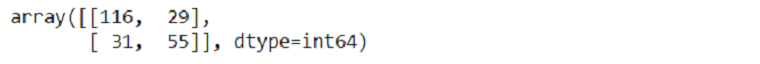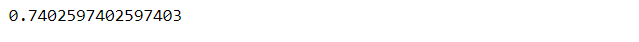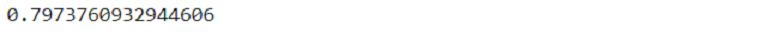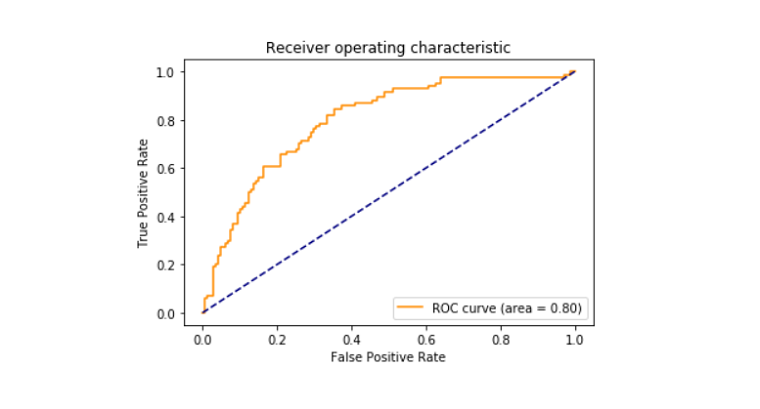• Category
• >Machine Learning

# What Is Naive Bayes Algorithm In Machine Learning?

• Rohit Dwivedi
• Apr 29, 2020
• Updated on: Jul 02, 2021## Introduction

Consider a case where you have created features, you know about the importance of features and you are supposed to make a classification model that is to be presented in a very short period of time?

What will you do? You have a very large volume of data points and very few features in the data set. In that situation, if I had to make such a model I would have used ‘Naive Bayes’, which is considered to be a really fast algorithm when it comes to classification tasks and will recommend it to you.

In this blog, I am trying to explain how the algorithm works that can be used in these kinds of scenarios, especially for binary and multiclass classification.

Naive Bayes is a machine learning model that is used for large volumes of data, even if you are working with data that has millions of data records the recommended approach is Naive Bayes. It gives very good results when it comes to NLP tasks such as sentimental analysis. It is a fast and uncomplicated classification algorithm.

To understand the naive Bayes classifier we need to understand the Bayes theorem. So let’s first discuss the Bayes Theorem.

## Bayes Theorem

It is a theorem that works on conditional probability. Conditional probability is the probability that something will happen, given that something else has already occurred. The conditional probability can give us the probability of an event using its prior knowledge.

### Conditional probability:Conditional Probability

Where,

P(A): The probability of hypothesis H being true. This is known as the prior probability.

P(B): The probability of the evidence.

P(A|B): The probability of the evidence given that hypothesis is true.

P(B|A): The probability of the hypothesis given that the evidence is true.

(Suggested read: Introduction to Bayesian Statistics)

## Naive Bayes Classifier

A classifier is a machine learning model segregating different objects on the basis of certain features of variables.

It is a kind of classifier that works on the Bayes theorem. Prediction of membership probabilities is made for every class such as the probability of data points associated with a particular class.

The class having maximum probability is appraised as the most suitable class. This is also referred to as Maximum A Posteriori (MAP).

• The MAP for a hypothesis is:

• 𝑀𝐴𝑃 (𝐻) = max 𝑃((𝐻|𝐸))

• 𝑀𝐴𝑃 (𝐻) = max 𝑃((𝐻|𝐸)  ∗ (𝑃(𝐻)) /𝑃(𝐸))

• 𝑀𝐴𝑃 (𝐻) = max(𝑃(𝐸|𝐻) ∗ 𝑃(𝐻))

• 𝑃 (𝐸) is evidence probability, and it is used to normalize the result. The result will not be affected by removing 𝑃(𝐸).

Naive Bayes classifiers conclude that all the variables or features are not related to each other. The Existence or absence of a variable does not impact the existence or absence of any other variable. For example,

• Fruit may be observed to be an apple if it is red, round, and about 4″ in diameter.

• In this case also even if all the features are interrelated to each other, an naive bayes classifier will observe all of these independently contributing to the probability that the fruit is an apple.

We experiment with the hypothesis in real datasets, given multiple features. So, computation becomes complex.

(Similar read: How to use the Random Forest classifier in Machine learning?)

### Types Of Naive Bayes Algorithms

1. Gaussian Naïve Bayes:  When characteristic values are continuous in nature then an assumption is made that the values linked with each class are dispersed according to Gaussian that is Normal Distribution.

2. Multinomial Naïve Bayes: Multinomial Naive Bayes is favored to use on data that is multinomial distributed. It is widely used in text classification in NLP.  Each event in text classification constitutes the presence of a word in a document.

3. Bernoulli Naïve Bayes:  When data is dispensed according to the multivariate Bernoulli distributions then Bernoulli Naive Bayes is used. That means there exist multiple features but each one is assumed to contain a binary value. So, it requires features to be binary-valued.

As discussing such statistical distribution, learn more about types of the statistical data distribution to know them in detail.

• It is a highly extensible algorithm that is very fast.

• It can be used for both binaries as well as multiclass classification.

• It has mainly three different types of algorithms that are GaussianNB, MultinomialNB, BernoulliNB.

• It is a famous algorithm for spam email classification.

• It can be easily trained on small datasets and can be used for large volumes of data as well.

• The main disadvantage of the NB is considering all the variables independent that contributes to the probability.

### Applications of Naive Bayes Algorithms

• Real-time Prediction:  Being a fast learning algorithm can be used to make predictions in real-time as well.

• MultiClass Classification:  It can be used for multi-class classification problems also.

• Text Classification: As it has shown good results in predicting multi-class classification so it has more success rates compared to all other algorithms. As a result, it is majorly used in sentiment analysis & spam detection.

### Hands-On Problem Statement

The problem statement is to classify patients as diabetic or non-diabetic. The dataset can be downloaded from the Kaggle website that is ‘PIMA INDIAN DIABETES DATABASE’. The datasets had several different medical predictor features and a target that is ‘Outcome’. Predictor variables include the number of pregnancies the patient has had, their BMI, insulin level, age, and so on.

``````import numpy as np
import pandas as pd
import seaborn as sns
sns.set(color_codes=True)
import matplotlib.pyplot as plt
%matplotlib inline
from sklearn.preprocessing import StandardScaler

from sklearn.model_selection import train_test_split
from sklearn.naive_bayes import GaussianNB
from sklearn import metrics

colnames = ['preg', 'plas', 'pres', 'skin', 'test', 'mass', 'pedi', 'age', 'class']

X = data.drop(Outcome”, axis = 1)
Y = data[ [“outcome”] ]
X_train, X_test, Y_train, Y_test = train_test_split(X,Y, test_size= 0.2, random_state = 1)

model = GaussianNB()
model.fit(X_train, Y_train)
Y_pred = model.predict(X_test)
``````

Code implementation of importing and splitting the data

STEPS-

• Initially, all the necessary libraries are imported like numpy, pandas, train-test_split, GaussianNB, metrics.
• Since it is a data file with no header, we will supply the column names that have been obtained from the above URL
• Created a python list of column names called "names".
• Initialized predictor variables and the target that is X and Y respectively.
• Transformed the data using StandardScaler.
• Split the data into training and test sets.
• Created an object for GaussianNB.
• Fitted the data in the model to train it.
• Made predictions on the test set and stored it in a ‘predictor’ variable.

For doing the exploratory data analysis of the dataset you can look for the techniques.

``````from sklearn import metrics

# make predictions
predicted = model.predict(X_test)
from sklearn.metrics import accuracy_score, confusion_matrix
metrics.confusion_matrix(predicted, y_test)
``````Confusion-matrix & model score test data

• Imported accuracy_score and confusion_matrix from sklearn.metrics. Printed the confusion matrix between predicted and actual that tells us the actual performance of the model.
• Calculated the model_score of the testing data to know how good is the model performing in generalizing the two classes which came out to be 74%.

``````model_score = model.score(X_test, y_test)
model_score
````````````y_predictProb = model.predict_proba(X_test)
from sklearn.metrics import auc, roc_curve
fpr, tpr, thresholds = roc_curve(y_test, y_predictProb[::,1])
roc_auc = auc(fpr, tpr)
roc_auc
``````• Imported auc, roc_curve again from sklearn.metrics.
• Printed roc_auc score between false positive and true positive that came out to be 79%.
• Imported matplotlib.pyplot library to plot the roc_curve.
• Printed the roc_curve.
``````plt.plot(fpr, tpr, color='darkorange', label='ROC curve (area = %0.2f)' % roc_auc)
plt.plot([0, 1], [0, 1], color='navy', linestyle='--')
plt.xlabel('False Positive Rate')
plt.ylabel('True Positive Rate')
plt.legend(loc="lower right")
``````Roc_curve

The receiver operating characteristic curve also known as roc_curve is a plot that tells about the interpretation potential of a binary classifier system. It is plotted between the true positive rate and the false positive rate at different thresholds. The ROC curve area was found to be 0.80.

(Also read: AUC-ROC Curve Tutorial: Working and Applications)

## Conclusion

Naive Bayes algorithms are widely deployed for sentiment analysis, spam filtering, recommendation systems etc. They are fast and easier to employ but have the biggest disadvantage “the requirement of predictors to be independent”.

In this blog, I have discussed Naive Bayes algorithms used for classification tasks in different contexts. I have discussed what is the role of Bayes theorem in NB Classifier, different characteristics of NB, advantages, and disadvantages of NB, application of NB, and in the last I have taken a problem statement from Kaggle that is about classifying patients as diabetic or not.

For the python file and also the used dataset in the above problem you can refer to the Github link here that contains both.